#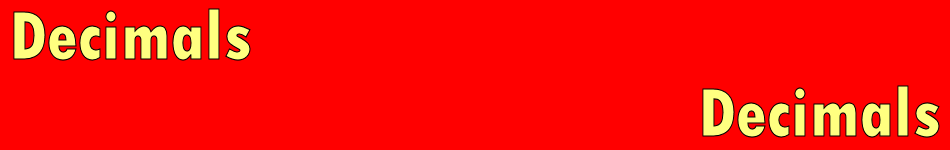There are 366 different Starters of The Day, many to choose from. You will find below some starters on the topic of Decimals. A lesson starter does not have to be on the same topic as the main part of the lesson or the topic of the previous lesson. It is often very useful to revise or explore other concepts by using a starter based on a totally different area of Mathematics.

Main Page

### Decimals Starters: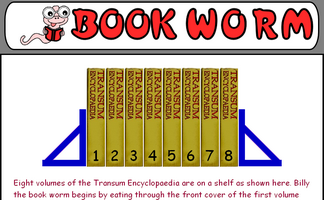Work out the distance the book worm needs to eat through to get to the back cover of the last book.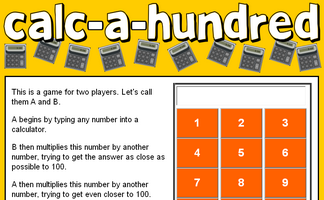A game for two players requiring a calculator and thinking skills.How old is a person if when her age is divided by certain numbers, the calculator display ending are as shown.Arrange the fractions and decimals in order from smallest to largest.Convert fractions to decimals, decimals to percentages and percentages to fractions.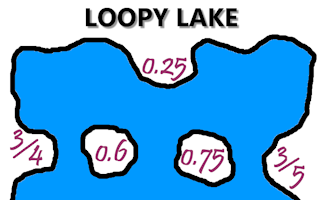Match the fractions to their decimal equivalents and join them with lines that do not cross.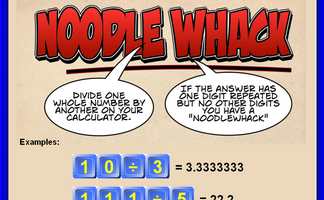Divide one whole number by another on your calculator. If the answer has one digit repeated but no other digits you have a Noodlewhack.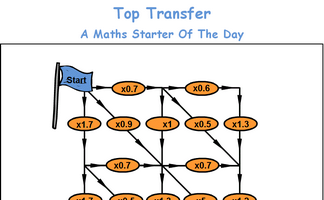Beginning with 100 on your calculator, what is the largest and smallest totals you can end up with after travelling through each of the possible routes.

## Exercises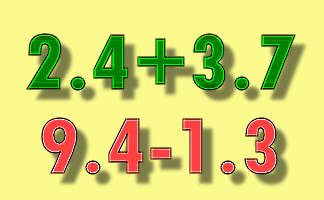#### Decimal Plus

Practise mental and written methods for adding and subtracting decimal numbers.#### Decimal Products

Find two decimal numbers that add up to exactly one. What is the product of these two decimals?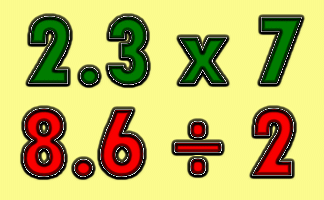#### Decimal Times

Practise mental and written methods for multiplying and dividing decimal numbers.#### Decimals Line

A number line showing tenths and hundredths with draggable arrows. This is a visual aid designed to be projected onto a whiteboard for whole class exposition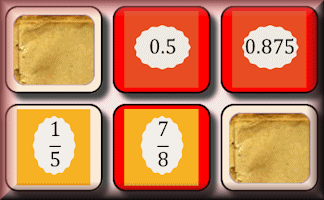#### Fraction Decimal Pairs

The traditional pairs or Pelmanism game adapted to test knowledge of simple fractions and their equivalent decimals.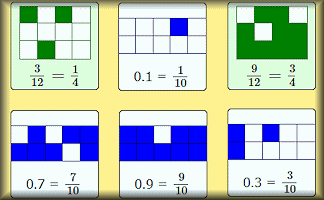#### Fraction Line

Arrange the fractions in order from smallest to largest.#### Fraction Order

Arrange the fractions and decimals in order from smallest to largest.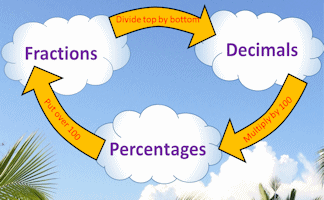#### Fractions Decimals Percentages

Revise the methods for converting fractions to decimals and percentages.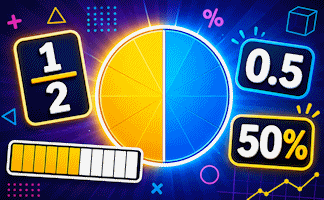#### Fractions, Decimals, Percentages

An exercise on converting fractions to decimals, decimals to percentages and percentages to fractions.#### Inequalities

Check that you know what inequality signs mean and how they are used to compare two quantities. Includes negative numbers, decimals, fractions and metric measures.#### Particular Pipes

Construct the pipes using a set number of pieces with lengths given as fractions, decimals or percentages.A self marking exercise on the reading of scales of different types.#### Recurring Decimals

Change recurring decimals into their corresponding fractions and vica versa.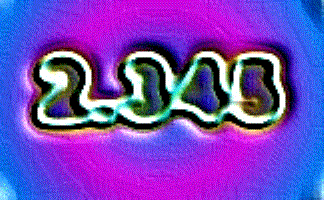#### Rounding SF

A self marking exercise requiring students to round numbers to a given number of significant figures.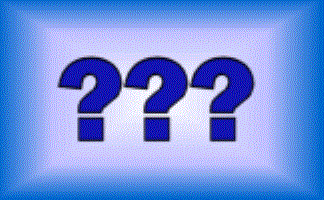#### Think of a Number

Ten students think of a number then perform various operations on that number. You have to find what the original numbers were.

### Search

The activity you are looking for may have been classified in a different way from the way you were expecting. You can search the whole of Transum Maths by using the box below.

Have today's Starter of the Day as your default homepage. Copy the URL below then select
Tools > Internet Options (Internet Explorer) then paste the URL into the homepage field.

Set as your homepage (if you are using Internet Explorer)

Do you have any comments? It is always useful to receive feedback and helps make this free resource even more useful for those learning Mathematics anywhere in the world. Click here to enter your comments.For All: